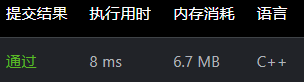# LeetCode316-去除重复字母：双HashMap计数字符串

```1 输入：s = "bcabc"
2 输出："abc"```

1.遍历统计各字符出现个数

2.再次遍历，先判断res中字符出现次数，再进行循环判断当前s[i]是否可以前向替换

``` 1 class Solution {
2 public:
3     string removeDuplicateLetters(string s) {
4         unordered_map<char,int>M;
5         unordered_map<char,int>N;
6         for(auto x:s)M[x]++;
7         string res="";
8         for(int i=0;i<s.size();i++){
9             if(N[s[i]]>0){
10                 M[s[i]]--;
11                 continue;
12             }
13             if(res.size()>=1){
14                while(res.size()>0&&M[res[res.size()-1]]!=0&&s[i]<res[res.size()-1]){//前向替换条件
15                 int n=res.size();
16                 N[res[n-1]]--;//注意先更新次数；替换后M[]不变；
17                 res.erase(n-1,1);
18               }
19             }
20             res+=s[i];
21             M[s[i]]--;
22             N[s[i]]++;
23     }
24         return res;
25     }
26 };```### 推荐这些技术文章：

acwing 基础算法课

AcWing算法基础课

acwing785
#include<iostream>
using namespace std;

const int N = 1e6 + 10;
int q[N];
int n;

void quick_sort(int q[], int l, int r) {
if(l >= r) return;
in...

1377. T 秒后青蛙的位置,unordered_map+bitset,学习复合类型关键字如何添加hash函数

bitset保存遍历状态
unordered_map保存dp值
unordered_map自定义键用法

#include<bits/stdc++.h>
#define pb push_back
using namespace std;
const int N=1e2+5;
class Solution {
public:
struct hash...

R语言中实现数据框或矩阵中字符串的批量替换

1、数据框或矩阵中字符串的批量替换

> a <- c("a","d", "v", "c")
> b <- c("c", "b", "a", "d")
> c <- c("m", "a", "d", "b")
> d <- data.frame(a, b, c)
> d
a b c
1 a c m
2 d b a
3 v a...

<P>&nbsp;</P>

...

if判断条件过多,利用Map,让代码表达更清晰

int a = 1;
if(a ==1 ||  a == 3 || a == 4 || a == 8 || a == 11){
}
=====================================
Map<Integer,Boolean> map = new HashMap();
map.put( 1, true);
map.put(...

LeetCode3. 无重复字符的最长子串

1 class Solution ...

1 class Solution {
2 public:
3 typedef pair<int,int> pii;
4 const int k1=1331;
5 const int k2=13331;
6 const int mod1=1e9+7;
7 const int mod2=1e9+9;
8 int p1[10...

821. 字符的最短距离

821. 字符的最短距离

1.使用HashMap
class Solution {
public int fourSumCount(int[] nums1, int[] nums2, int[] nums3, int[] nums4) {
//统计前两个数组中的元素之和(key)，以及出现次数(valuevalue)
Map<Integer,Integer> map =...

c++/c 中的字符串sring/字符串数组char [] 是如何比较大小的

c++中的string
(1)从第一位开始，依次比较两个字符串中每个字符对应ASCII码的大小，大的字符串大。
string a="abcd5sdse";
string b="er3dse";

​ b > a，
(2)若b为a的前一部分，则a >= b
string a="2541";
string b="25";

​ a > b
c中的 char []数组
#incl...

THE END